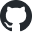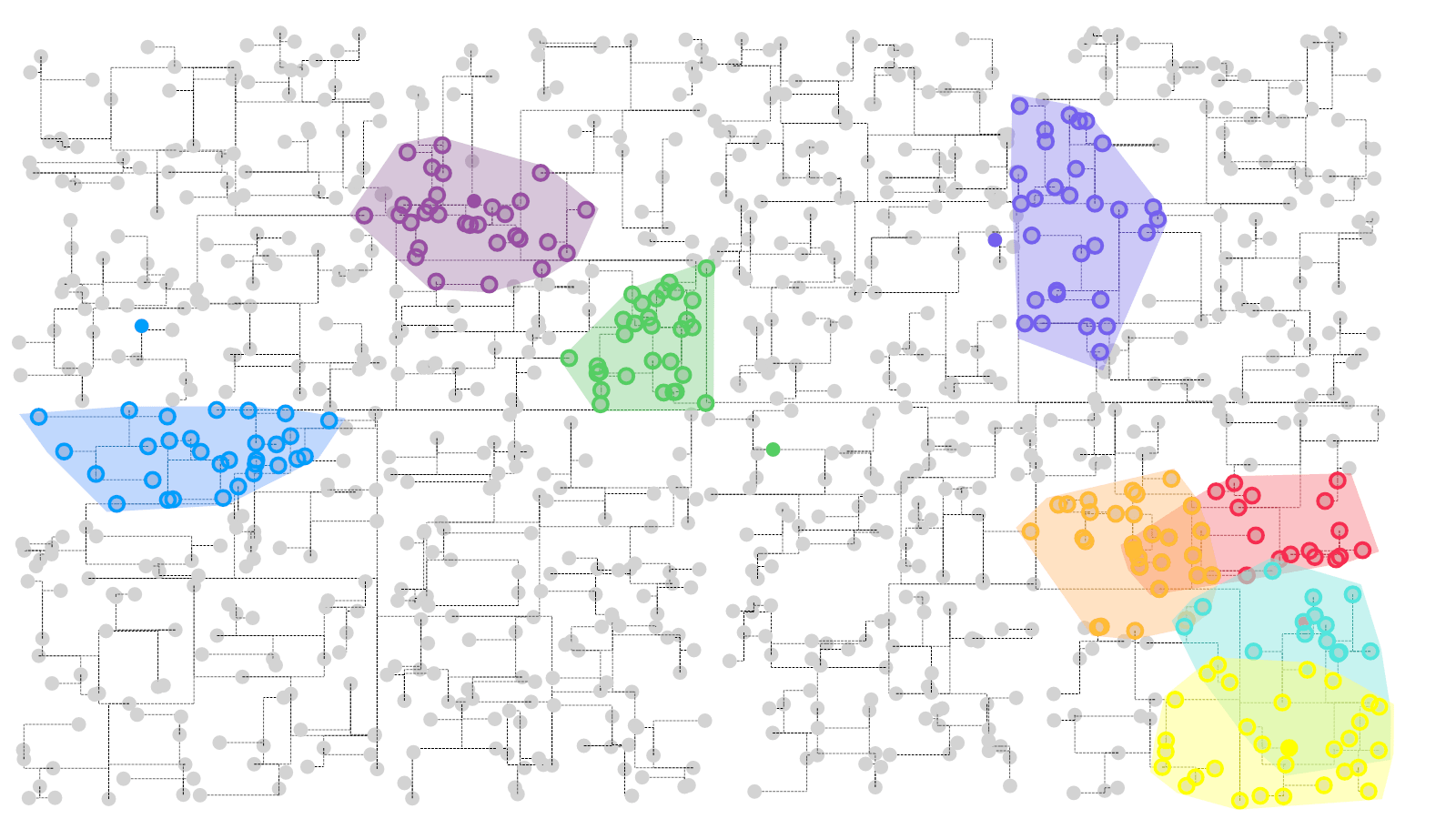https://github.com/xieguigang/sciBASIC## KD树与K邻近点计算• 则我们最开始可以先对X维度做二分分割：在上图中的root节点中，X等于7，所以我们可以将所有X小于7的数据点都放到左边，X大于7的数据点都放到右边。这样子就构建出了一个以X维度为key的二叉树。
• 接着呢，我们继续分别对左边以及右边所划分出来的数据点做Y维度的二分分割：以左边的数据点为例，第二排的数据点中，Y维度的值为4，所以我们可以将左边的数据中，Y小于4的都继续放到左边，Y大于4的数据点都放到右边
• 这样子呢，我们只需要递归的重复执行上面的两个步骤，就可以对数据点中的每一个维度都建立了二叉树。这个时候，我们所建立的二叉树就是KD树。Private Function buildTree(points As T(), depth As Integer, parent As KdTreeNode(Of T)) As KdTreeNode(Of T)
Dim axis = depth Mod dimensions.Length
Dim median As Integer
Dim node As KdTreeNode(Of T)

If points.Length = 0 Then
Return Nothing
ElseIf points.Length = 1 Then
Return New KdTreeNode(Of T)(points(Scan0), axis, parent)
Else
' sort by the axis dimensions
points.Sort(Function(a, b)
Return access(a, dimensions(axis)) - access(b, dimensions(axis))
End Function)

_counts += 1
median = stdNum.Floor(points.Length / 2)
End If

Dim left = points.slice(0, median).ToArray
Dim right = points.slice(median + 1).ToArray

node = New KdTreeNode(Of T)(points(median), axis, parent)
node.left = buildTree(left, depth + 1, node)
node.right = buildTree(right, depth + 1, node)

Return node
End Function

Dim left = points.slice(0, median).ToArray
Dim right = points.slice(median + 1).ToArray

## KD树查找

Dim dimension As Integer = depth Mod dimensions.Length
Dim axis As String = dimensions(dimension)

' whats got the got best _search result? left or right?
Dim goLeft = access(node.data, axis) < access(point.data, axis)
Dim target = If(goLeft, node.left, node.right)
Dim opposite = If(goLeft, node.right, node.left)

' target has our most likely nearest point, we go down that side of the
' tree first
If Not target Is Nothing Then
Call nearestSearch(point, target, depth + 1, result, maxNodes)
End If

' _search the opposite direction, only if there is potentially a better
' value than the longest distance we already have in our _search results
If Not opposite Is Nothing AndAlso opposite.distanceSquared(point.data, access) <= result(result.Count - 1).distance Then
Call nearestSearch(point, opposite, depth + 1, result, maxNodes)
End If

## KD树可视化

Dim size As New Size(3600, 2700)
Dim points2 As Point2D() = 1000 _
.SeqRandom _
.Select(Function(i)
New Point2D(randf.NextInteger(size.Width), randf.NextInteger(size.Height))
End Function) _
.ToArray
Dim tree As New KdTree(Of Point2D)(points2, New PointAccess)
Dim query = {
New NamedValue(Of PointF)("1", points2.Random, "#009EFB"),
New NamedValue(Of PointF)("1", points2.Random, "#55CE63"),
New NamedValue(Of PointF)("1", points2.Random, "#F62D51"),
New NamedValue(Of PointF)("1", points2.Random, "#FFBC37"),
New NamedValue(Of PointF)("1", points2.Random, "#7460EE"),
New NamedValue(Of PointF)("1", points2.Random, "#52E5DD"),
New NamedValue(Of PointF)("1", points2.Random, "#984ea3"),
New NamedValue(Of PointF)("1", points2.Random, "#ffff00")
}

Call DrawKDTree _
.Save("/path/top/demo_visual.png")### Order by Date Name Attachments

1.2.5.